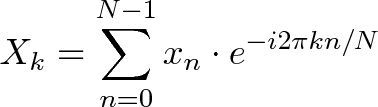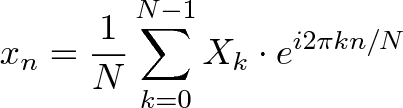# Understanding the Fourier transform

The Fourier transform is a mathematical technique that allows an MR signal to be decomposed into a sum of sine waves of different frequencies, phases, and amplitudes. This remarkable result derives from the work of Jean-Baptiste Joseph Fourier (1768-1830), a French mathematician and physicist.  Since spatial encoding in MR imaging involves frequencies and phases, it is naturally amenable to analysis by Fourier techniques.

The Fourier Transform is one of deepest insights ever made. Unfortunately, the meaning is buried within dense equations:$\displaystyle{X_k = \sum_{n=0}^{N-1} x_n \cdot e^{-i 2 \pi k n / N}}$$\displaystyle{x_n = \frac{1}{N} \sum_{k=0}^{N-1} X_k \cdot e^{i 2 \pi k n / N}}$

The Fourier transform relates the function’s time domain, shown in red, to the function’s frequency domain, shown in blue. The component frequencies, spread across the frequency spectrum, are represented as peaks in the frequency domain. Here is what Fourier transform does:Kudos to Lucas V. Barbosa to release the public domain such awesome animations (Wiki source)

In this post you can find some useful links explaining Fourier Transforms, as well as the FFT. Books, posts, videos and tutorials in MATLAB and Python will help you understand the general idea of the Fourier transform.

### Books

• Analyzing Neural Time-series Data by Micheal X. Cohen.

This book offers a comprehensive guide to the theory and practice of analyzing electrical brain signals. It explains the conceptual, mathematical, and implementational (via Matlab programming) aspects of time-, time-frequency- and synchronization-based analyses of magnetoencephalography (MEG), electroencephalography (EEG), and local field potential (LFP) recordings from humans and nonhuman animals. It is the only book on the topic that covers both the theoretical background and the implementation in language that can be understood by readers without extensive formal training in mathematics, including cognitive scientists, neuroscientists, and psychologists.

As an alternative, you can check Cohen’s lecture about the Fourier transform here.

### Blog/Website

• “What is an intuitive way of explaining how the Fourier transform works?”

A Quora post with some great answers on the intuition behind the Fast Fourier Transform. The first explanation is fantastic and full of history and detail.

• An Interactive Guide To The Fourier Transform.

Rather than jumping into the symbols, BetterExplained people experience the key idea firsthand by using a “smoothie” metaphor.

• Understanding the Fourier transform

Blog written by Stuart Riffle that gives an intuitive way to picture the Fourier transform based on his own experience at the library.

• Understanding the FFT algorithm

post on FFT from Jake Vanderplas is also a great explanation of how it works. Moreover, it can also be used a Python tutorial for FFT beginners.

• The beauty of computational efficiency: The Fast Fourier Transform

Predictably Noisy writes a post with Python commands to see what the FFT’s efficiency looks like.

### Videos

• How to do a Fast Fourier Transform (FFT) with Correct Amplitude Output in Matlab

In this tutorial, we will go over how to do a fast Fourier transform on a time domain signal properly using Matlab. Since Matlab’s FFT function does not provide an intuitive amplitude or frequency interval, we have developed a free code to do this for you. The amplitude units of the FFT returned by our function is the same as the amplitude units of the input signal.

• Introduction to the Fourier transform

This video is an introduction to the Fourier Transform. The author, Brian Douglas, tries to give a little bit of background into what the transform does and then he goes step by step through explaining the Inverse Transform in detail. He meant to cover the entire topic in this video but he ran of time so now there will be a part 2 which cover the Forward Fourier Transform.

### References

Featured image source.